In this post, common machine learning techniques, such as feature engineering, data transformation, Cross validation, and hyperparameter tuning are applied to a several regression-based and tree-based classifiers. For this comparative analysis the Covertype dataset from UCI machine learning repository is used to predict the type of forest coverage from one of the 7 categories. This is a single-label multi-class classification with equal weight classes.
For this work we selected the following multi-class classifiers:

1. Logistic Regression
2. Ridge Regression
3. Random Forest
5. XGBoost
• Dataset information: Researchers at the Department of Forest Sciences at Colorado State University collected over half a million measurements from tree observations from four areas of the Roosevelt National Forest in Colorado. All observations are cartographic variables (no remote sensing) from 30-meter x 30-meter sections of forest. The resulting dataset includes information on tree type, shadow coverage, distance to nearby landmarks (roads etcetera), soil type, and local topography. This dataset was obtained from UCI machine learning repository. It contains 15120 observations and 56 columns in total. This data includes 7 categories of different cover types, which will be used as the target label for prediction, 10 continuous variables, and 2 categorical variables, Wilderness areas and soil types. The categorical variables are encoded with one hot encoder.

Bache, K. & Lichman, M. (2013). UCI Machine Learning Repository. Irvine, CA: University of California, School of Information and Computer Science

``````# Import data
import pandas as pd
import numpy as np

print(data.shape)
``````

(15120, 56)

Id Elevation Aspect Slope Horizontal_Distance_To_Hydrology Vertical_Distance_To_Hydrology Horizontal_Distance_To_Roadways Hillshade_9am Hillshade_Noon Hillshade_3pm Horizontal_Distance_To_Fire_Points Wilderness_Area1 Wilderness_Area2 Wilderness_Area3 Wilderness_Area4 Soil_Type1 Soil_Type2 Soil_Type3 Soil_Type4 Soil_Type5 Soil_Type6 Soil_Type7 Soil_Type8 Soil_Type9 Soil_Type10 Soil_Type11 Soil_Type12 Soil_Type13 Soil_Type14 Soil_Type15 Soil_Type16 Soil_Type17 Soil_Type18 Soil_Type19 Soil_Type20 Soil_Type21 Soil_Type22 Soil_Type23 Soil_Type24 Soil_Type25 Soil_Type26 Soil_Type27 Soil_Type28 Soil_Type29 Soil_Type30 Soil_Type31 Soil_Type32 Soil_Type33 Soil_Type34 Soil_Type35 Soil_Type36 Soil_Type37 Soil_Type38 Soil_Type39 Soil_Type40 Cover_Type
0 1 2596 51 3 258 0 510 221 232 148 6279 1 0 0 0 0 0 0 0 0 0 0 0 0 0 0 0 0 0 0 0 0 0 0 0 0 0 0 0 0 0 0 0 1 0 0 0 0 0 0 0 0 0 0 0 5
1 2 2590 56 2 212 -6 390 220 235 151 6225 1 0 0 0 0 0 0 0 0 0 0 0 0 0 0 0 0 0 0 0 0 0 0 0 0 0 0 0 0 0 0 0 1 0 0 0 0 0 0 0 0 0 0 0 5
2 3 2804 139 9 268 65 3180 234 238 135 6121 1 0 0 0 0 0 0 0 0 0 0 0 0 0 0 1 0 0 0 0 0 0 0 0 0 0 0 0 0 0 0 0 0 0 0 0 0 0 0 0 0 0 0 0 2
3 4 2785 155 18 242 118 3090 238 238 122 6211 1 0 0 0 0 0 0 0 0 0 0 0 0 0 0 0 0 0 0 0 0 0 0 0 0 0 0 0 0 0 0 0 0 1 0 0 0 0 0 0 0 0 0 0 2
4 5 2595 45 2 153 -1 391 220 234 150 6172 1 0 0 0 0 0 0 0 0 0 0 0 0 0 0 0 0 0 0 0 0 0 0 0 0 0 0 0 0 0 0 0 1 0 0 0 0 0 0 0 0 0 0 0 5

All the columns have int dtype. * The dataset has 15120 rows and 56 columns. This is a multiclass classification with “Cover_Type” as the target label. Wilderness_Area and Soil_Type columns have been encoded with One Hot Encoder. For better visualization, we will convert all the Soil_Types and Wilderness_Areas columns into two categorical features “Soil_Type” and “Wilderness_Area”.

``````data1 = data.copy()
# Encode Soil_Types and Wilderness_Area features into two new features
ohe_bool = []
enc_cols = ["Wilderness_Area", "Soil_Type"]
for idx, val in enumerate(enc_cols):
val_df = data.filter(regex=val, axis=1)
print(f"{idx} {val} is ohe? {((val_df.sum(axis=1)) == 1).all()}")
data1[val] = val_df.dot(val_df.columns)
# Convert the constructed columns into int
data1[val] = data1[val].astype('str').str.findall(r"(\d+)").str[-1].astype('int')
data1 = data1.drop(val_df.columns, axis=1)

# Reorder the columns
data1 = data1.iloc[:,data1.columns!="Cover_Type"].merge(data1["Cover_Type"], left_index=True, right_index=True)
``````

(15120, 14)

0 1 2596 51 3 258 0 510 221 232 148 6279 1 29 5
1 2 2590 56 2 212 -6 390 220 235 151 6225 1 29 5
2 3 2804 139 9 268 65 3180 234 238 135 6121 1 12 2
3 4 2785 155 18 242 118 3090 238 238 122 6211 1 30 2
4 5 2595 45 2 153 -1 391 220 234 150 6172 1 29 5
``````data1.describe()
``````
count 15120.00000 15120.000000 15120.000000 15120.000000 15120.000000 15120.000000 15120.000000 15120.000000 15120.000000 15120.000000 15120.000000 15120.000000 15120.000000 15120.000000
mean 7560.50000 2749.322553 156.676653 16.501587 227.195701 51.076521 1714.023214 212.704299 218.965608 135.091997 1511.147288 2.800397 19.171362 4.000000
std 4364.91237 417.678187 110.085801 8.453927 210.075296 61.239406 1325.066358 30.561287 22.801966 45.895189 1099.936493 1.119832 12.626960 2.000066
min 1.00000 1863.000000 0.000000 0.000000 0.000000 -146.000000 0.000000 0.000000 99.000000 0.000000 0.000000 1.000000 1.000000 1.000000
25% 3780.75000 2376.000000 65.000000 10.000000 67.000000 5.000000 764.000000 196.000000 207.000000 106.000000 730.000000 2.000000 10.000000 2.000000
50% 7560.50000 2752.000000 126.000000 15.000000 180.000000 32.000000 1316.000000 220.000000 223.000000 138.000000 1256.000000 3.000000 17.000000 4.000000
75% 11340.25000 3104.000000 261.000000 22.000000 330.000000 79.000000 2270.000000 235.000000 235.000000 167.000000 1988.250000 4.000000 30.000000 6.000000
max 15120.00000 3849.000000 360.000000 52.000000 1343.000000 554.000000 6890.000000 254.000000 254.000000 248.000000 6993.000000 4.000000 40.000000 7.000000

Next we plot the Heatmap of correlation matrix. It shows Elevation and Soil_Type are highly correlated, and Hillshade_3pm is reversely correlated with Hillshade_9am.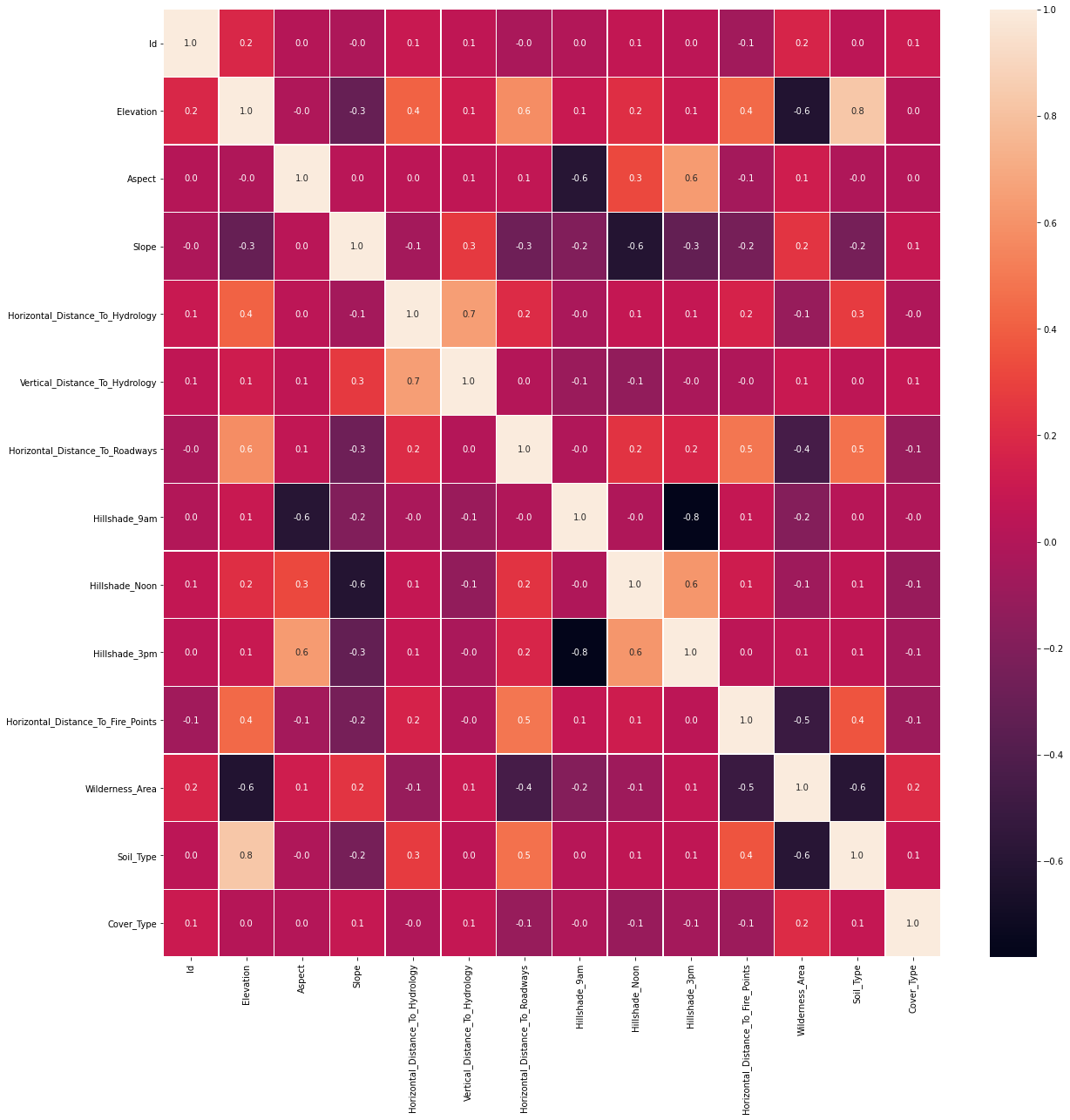Looking at scatter plot of different features, it seems “Elevation” is an important parameter in separating different classes of target label.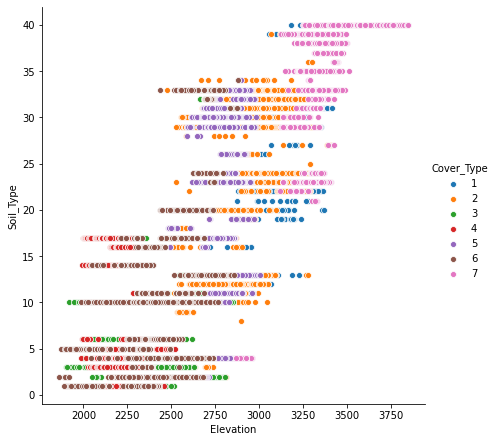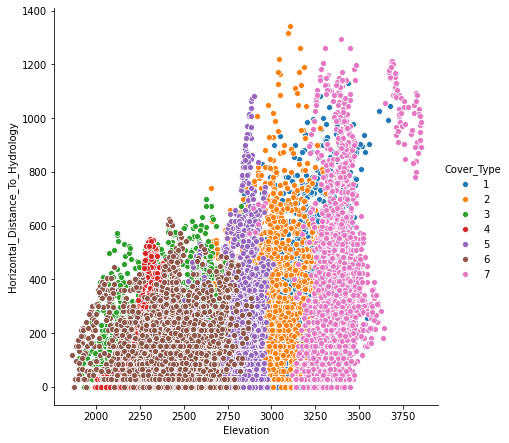We make the following observations from the Count plots below.

• Soil_Type10 shows a significant class distinction for Cover_Type=6.
• Similarly Wilderness_Area4 and Cover_Type=4 are strongly associated.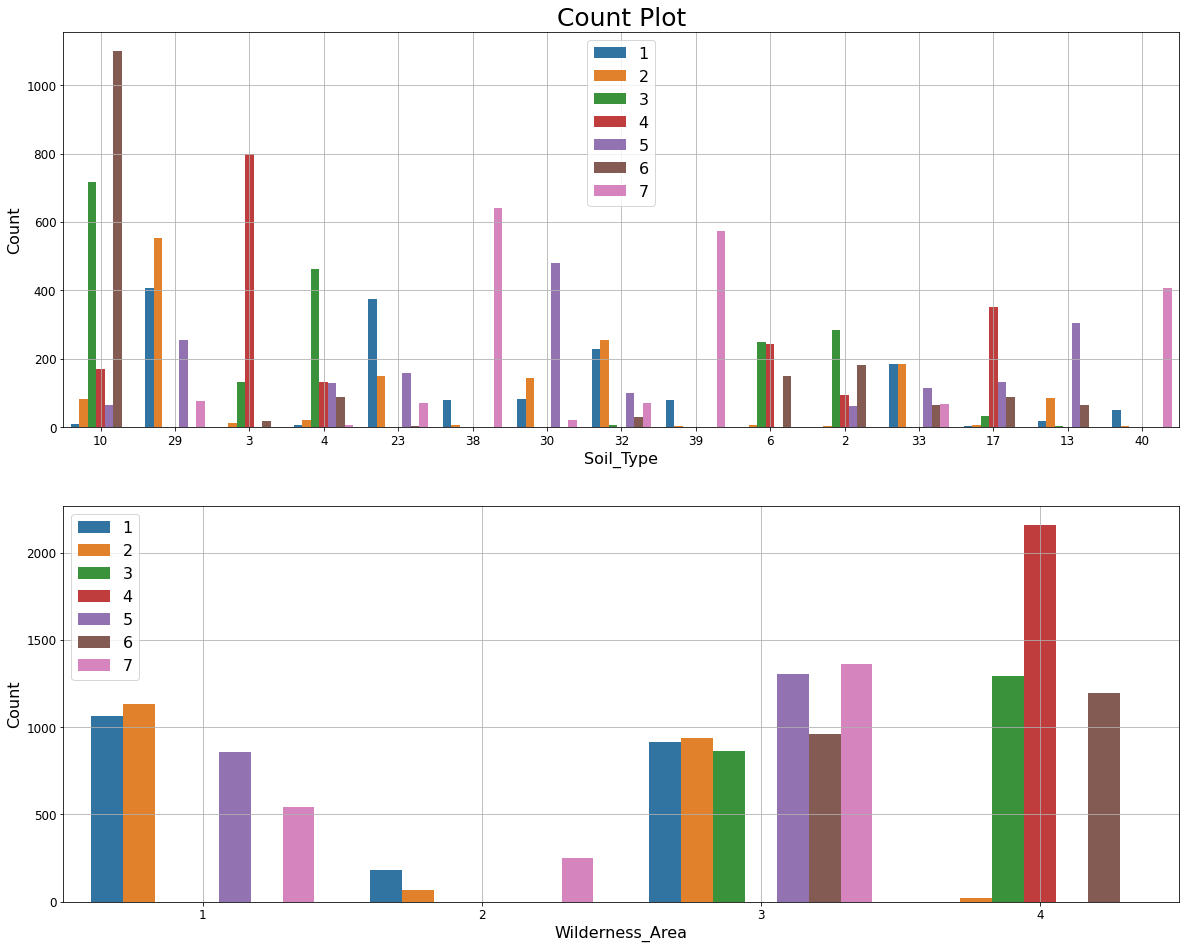List of unique values in the feature shows Soil_Type15 and Soil_Type7 are constant. Additionally, Id column is a unique identifier for each observation. We’ll drop those three columns as they do not carry any information to identify the target label. There are also skewness in some features. “Horizontal_Distance_To_Hydrology” is an example of that.### Train-Test Split

After removing “Id” and constant columns, data is split into train and validation set.

``````from sklearn.model_selection import train_test_split
# Split train into train & val
train, val = train_test_split(data, train_size=0.80, test_size=0.20, stratify=data["Cover_Type"], random_state=42)
# Separate class label and data
y_train = train["Cover_Type"]
X_train = train.drop("Cover_Type", axis=1)
y_val = val["Cover_Type"]
X_val = val.drop("Cover_Type", axis=1)
print(f'train: {train.shape}, val: {val.shape}')
``````

train: (12096, 56), val: (3024, 56)

### Baseline model

Normalized value counts of the target label shows an equal weight distribution for all classes, with baseline prediction of 14.3%.

### Pipeline Estimators, Feature_importances and permutation_importance

We are going to look at five different classifiers to compare their performance. For the regression classifiers we will standardize the data before fitting.

1. LogesticRegression
2. RidgeClassifier
3. RandomForestClassifier
5. XGBCLassifier

The following plots show the feature_importances and permutation importances of the tree-base classifiers. “Elevation” is given a higher importance weight compared to other features, as we saw earlier. Without the help of permutation, XGBClassifier does not seem to detect the importance of “Elevation” feature.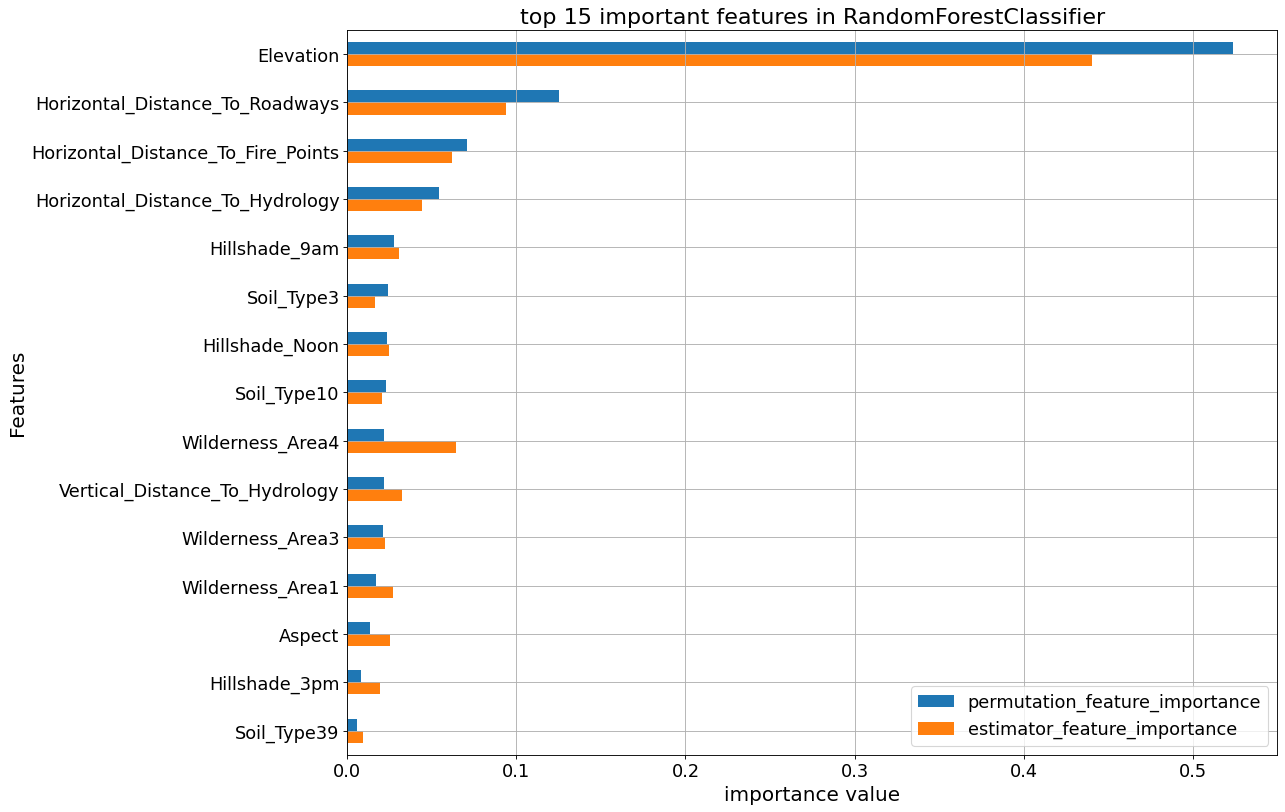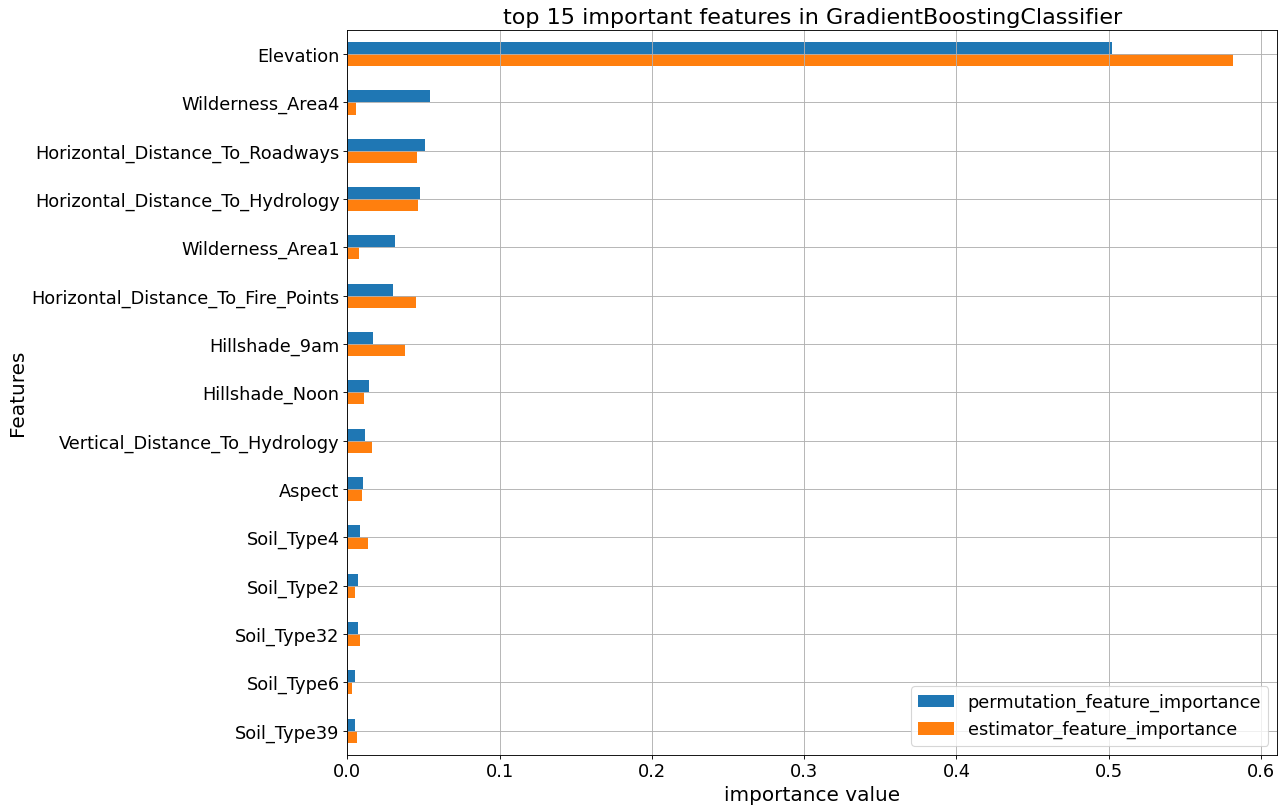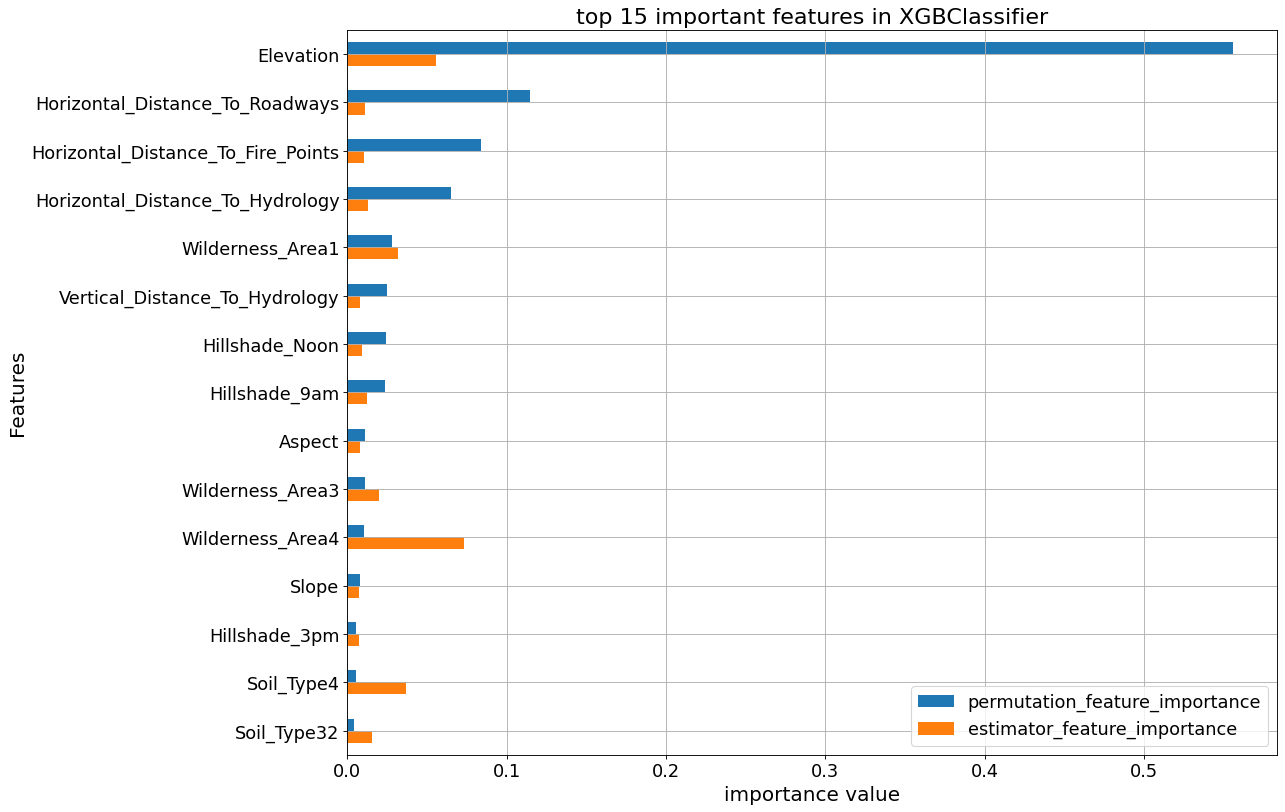### Avoid Overfitting By Early Stop feature in XGBoost Classifier

XGBClassifier tends to overfit and its growth parameters need to be controlled. To demonstrate that, an early stop fitting with 50 rounds is run below. We notice at some point in fitting process the validation error stops decreasing steady.

``````# XGBoost early stop fit
xform = make_pipeline(
FunctionTransformer(wrangle, validate=False),
# ce.OrdinalEncoder(),
)

xform.set_params(functiontransformer__kw_args = kwargs_dict)

X_train_xform = xform.fit_transform(X_train)
X_val_xform = xform.transform(X_val)

clf = XGBClassifier(
n_estimators = 1000,
max_depth=8,
learning_rate=0.5,
num_parallel_tree = 10,
n_jobs=-1
)

#eval_set
eval_set = [(X_train_xform, y_train), (X_val_xform, y_val)]

clf.fit(X_train_xform, y_train,
eval_set=eval_set,
eval_metric=['merror', 'mlogloss'],
early_stopping_rounds=50,
verbose=False) # Stop if the score hasn't improved in 50 rounds

print('Training Accuracy:', clf.score(X_train_xform, y_train))
print('Validation Accuracy:', clf.score(X_val_xform, y_val))
``````

Training Accuracy: 0.9987599206349206 Validation Accuracy: 0.8528439153439153

XGBoost trains upto 0.999 and yields 0.85 validation accuracy. The following graph shows how validation error remains constant while training error reduces.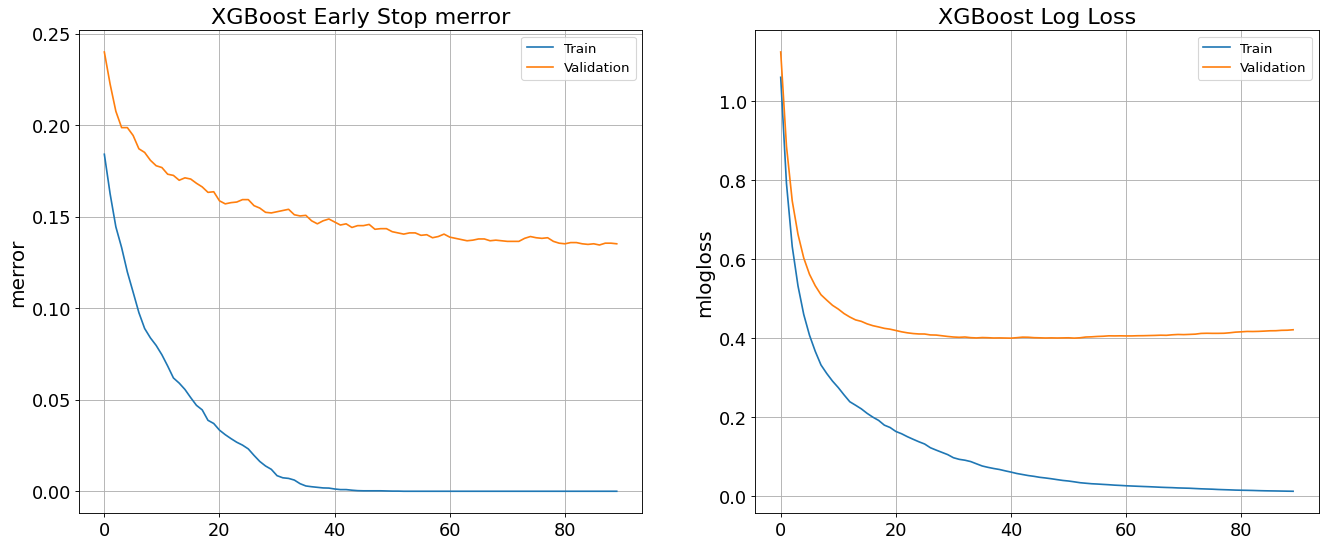### Cross Validation Curve

The training set is split into kfolds and the model is cross validated based on a classifier parameter.

``````kfold=4
for k, estimator in enumerate(tree_list):
print(f"********** {est_dict[estimator].__class__.__name__} **********")
classifier_name = clf_name(estimator, est_dict)
# estimator.set_params(functiontransformer__kw_args = kwargs_dict)
# print(est_dict[estimator])

param_distributions = {classifier_name.lower()+'__'+ est_dict[estimator][j]: est_dict[estimator][j] for j in range(len(est_dict[estimator]))}

for i in range(len(est_dict[estimator])):
param_name=classifier_name.lower()+'__'+ est_dict[estimator][i]
param_range = est_dict[estimator][i]
estimator.set_params(functiontransformer__kw_args = kwargs_dict)

train_scores, val_scores = validation_curve(estimator, X_train, y_train,
# param_name='functiontransformer__kw_args',
param_name=param_name,
param_range=param_range,
scoring='accuracy', cv=kfold, n_jobs=-1, verbose=0
)

print(f'**** {param_name} ****\n')
print("val scores mean:\n", np.mean(val_scores, axis=1))

# Averaging CV scores
fig, ax = plt.subplots(figsize=(12,8))

ax.plot(param_range, np.mean(train_scores, axis=1), color='blue', label='mean training accuracy')
ax.plot(param_range, np.mean(val_scores, axis=1), color='red', label='mean validation accuracy')
ax.set_title(f'Cross Validation with {kfold:d} folds', fontsize=20)
ax.set_xlabel(param_name, fontsize=18)
ax.set_ylabel('model score: Accuracy', fontsize=18)
ax.legend(fontsize=12)
ax.tick_params(axis='both', labelsize=16)
ax.grid(which='both')
``````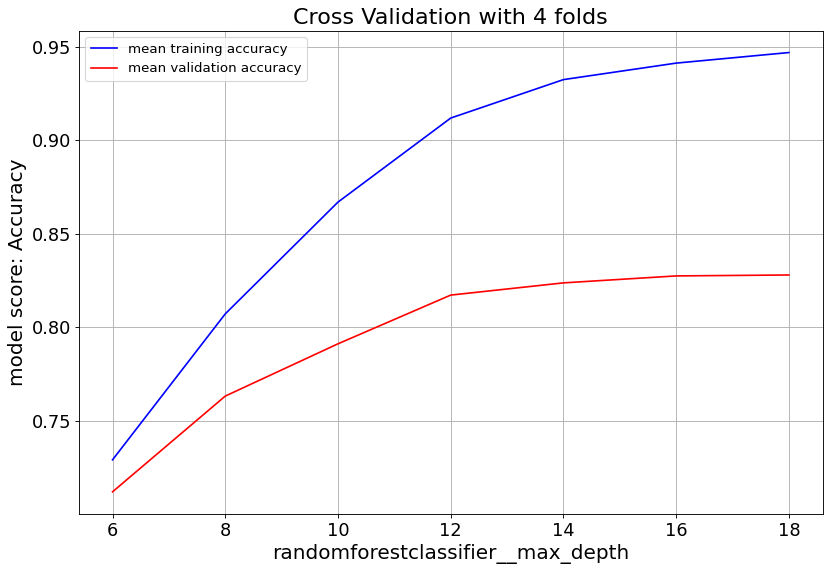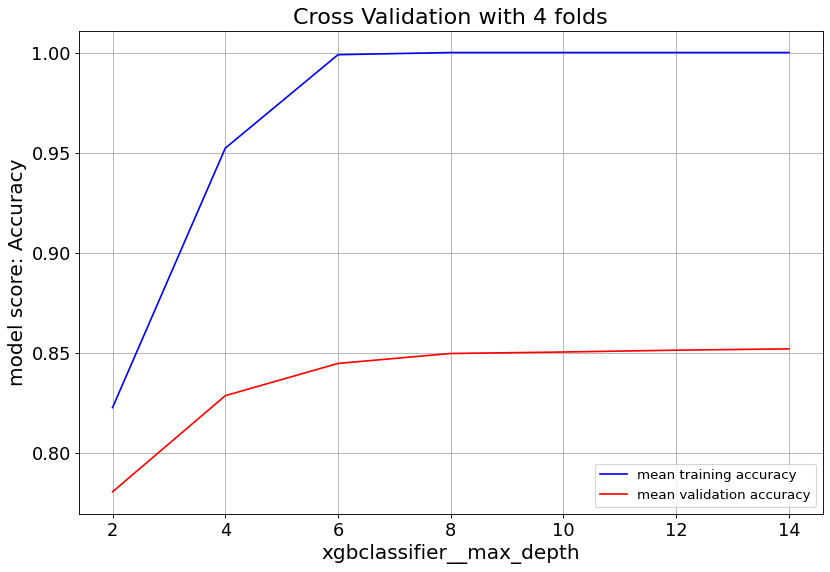Gradient Boost classifier family quickly overfit at max_depth>6. So it’s important to keep the tree depth shallow.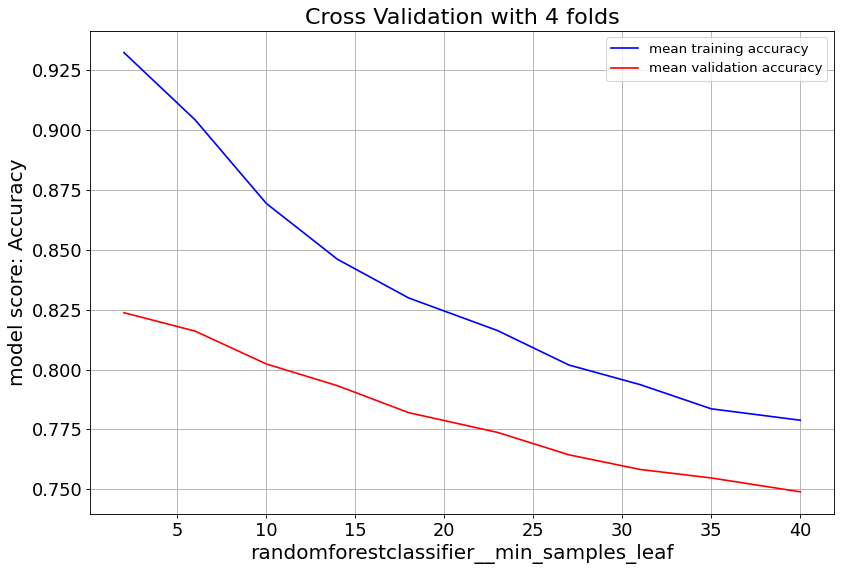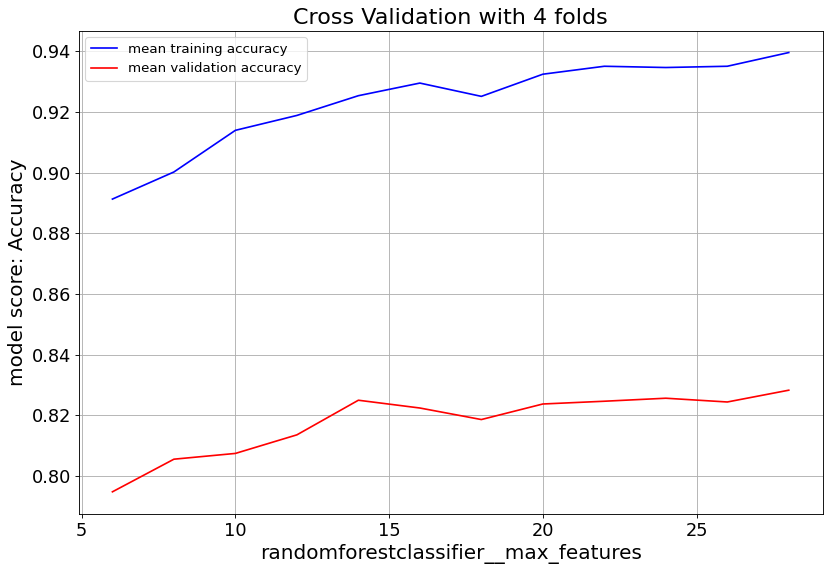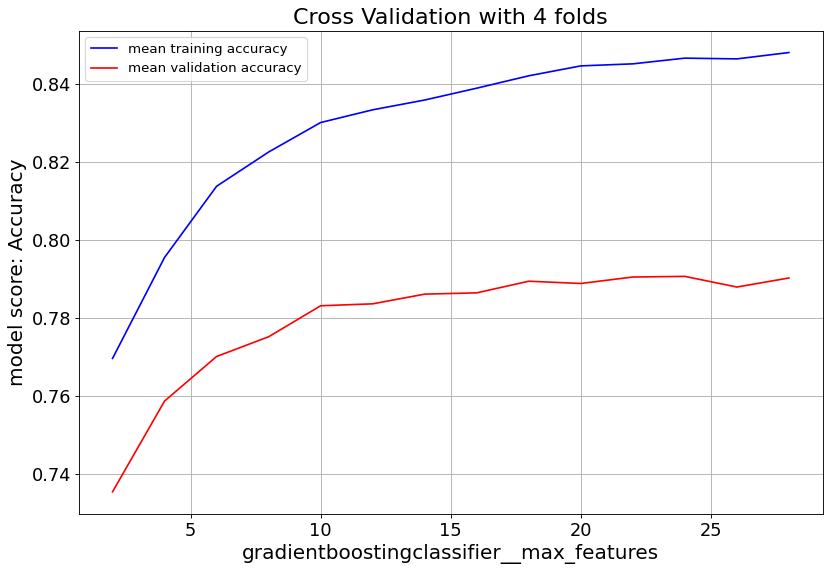max_feat parameter shows validation score saturates at numbers above 20, and starts to overfit. Lowering min_samples_leaf improves validation score. Hence we consider small numbers.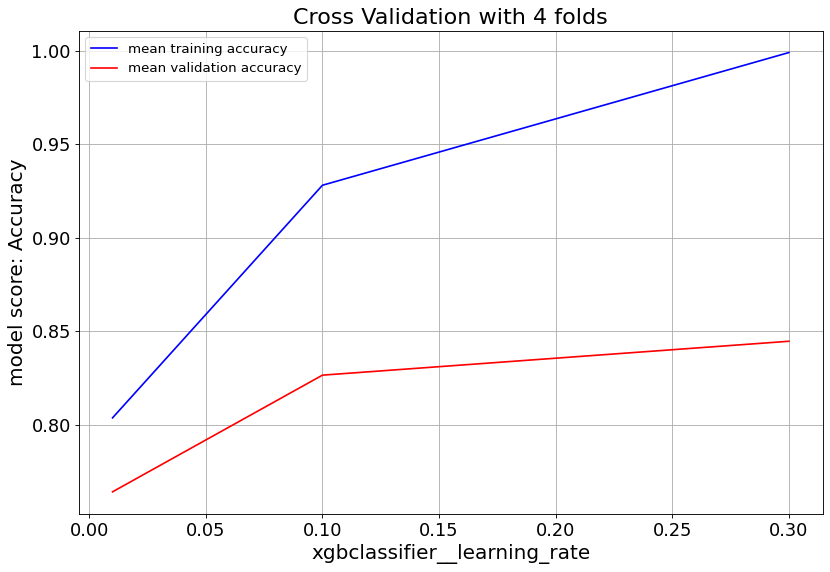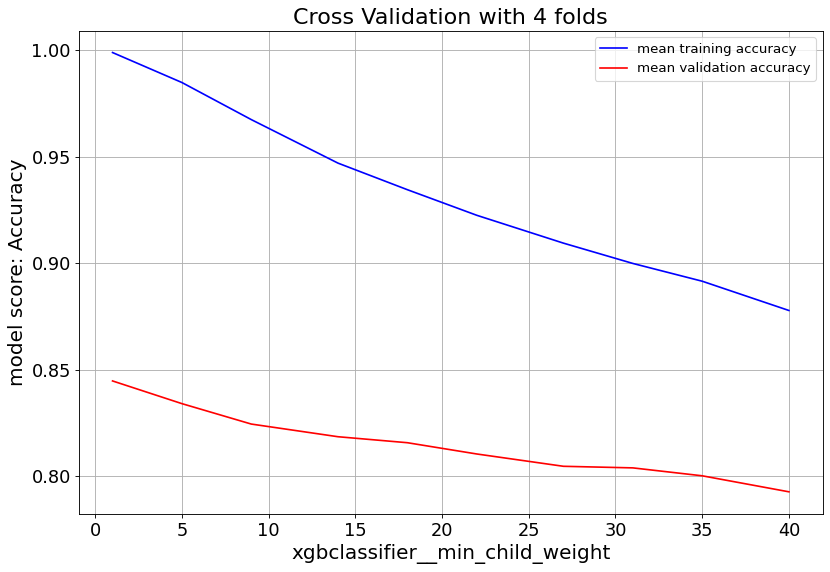For XGBoost we can slow down the overfitting issue with min_child_weight and learningrate parameters.

### Hyper Parameter Tunning

The parameters of all five estimators are optimized by RandomizedSearchCV.

``````kfold=4
n_iter = 10
best_ests = [np.NaN]*len(est)
best_scores = [np.NaN]*len(est)
best_params = [np.NaN]*len(est)

for i, estimator in enumerate(est):
print(f"\n********** {est_dict[estimator].__class__.__name__} **********")
estimator.set_params(functiontransformer__kw_args = kwargs_dict)

classifier_name = clf_name(estimator=estimator, est_dict=est_dict)
print(est_dict[estimator])

param_distributions = {classifier_name.lower()+'__'+ est_dict[estimator][j]:
est_dict[estimator][j] for j in range(len(est_dict[estimator]))}

rscv = RandomizedSearchCV(estimator, param_distributions=param_distributions,
n_iter=n_iter, cv=kfold, scoring='accuracy', verbose=1, return_train_score=True,
n_jobs=-1)

rscv.fit(X_train, y_train)
best_ests[i] = rscv.best_estimator_
best_scores[i] = rscv.best_score_
best_params[i] = rscv.best_params_

best_ypreds = [best_est.predict(X_val) for best_est in best_ests]
best_testscores = [accuracy_score(y_val, best_ypred) for best_ypred in best_ypreds]
print('Test accuracy\n', best_testscores)
print('best cross validation accuracy\n', best_scores)
``````

Test accuracy [0.6911375661375662, 0.6326058201058201, 0.7754629629629629, 0.8700396825396826, 0.8498677248677249] best cross validation accuracy [0.7080026455026454, 0.6332671957671958, 0.7709986772486773, 0.8645833333333334, 0.8445767195767195]

The accuracy scores above corresponds to LogisticRegression, RidgeClassifier RandomForestClassifier, GradientBoostingClassifier, XGBClassifier accordingly. In this work, GradientBoostingClassifier with 87% accuracy on the test data shows the best performance among the five.

## Conclusion

This post evaluates and compares the performance of five multi-class classifiers to predict the “Cover-Type” label of Covertype dataset. The dataset has 54 features, and one target label with 7 different classes. Target label classes are distributed evenly with baseline prediction of 14%. We splitted the total 15120 obeservations into train and test subsets. By applying commonly used classification methods such as feature selection, data scaling, cross validation, and hyperparameter optimization, we were able to achive accuracy scores ranging from 63% to 86% on test data subset. The score metrics of a typical fit of those classifiers has been deployed onto heroku.